Electronics

# Proper IC interconnects for high-speed signaling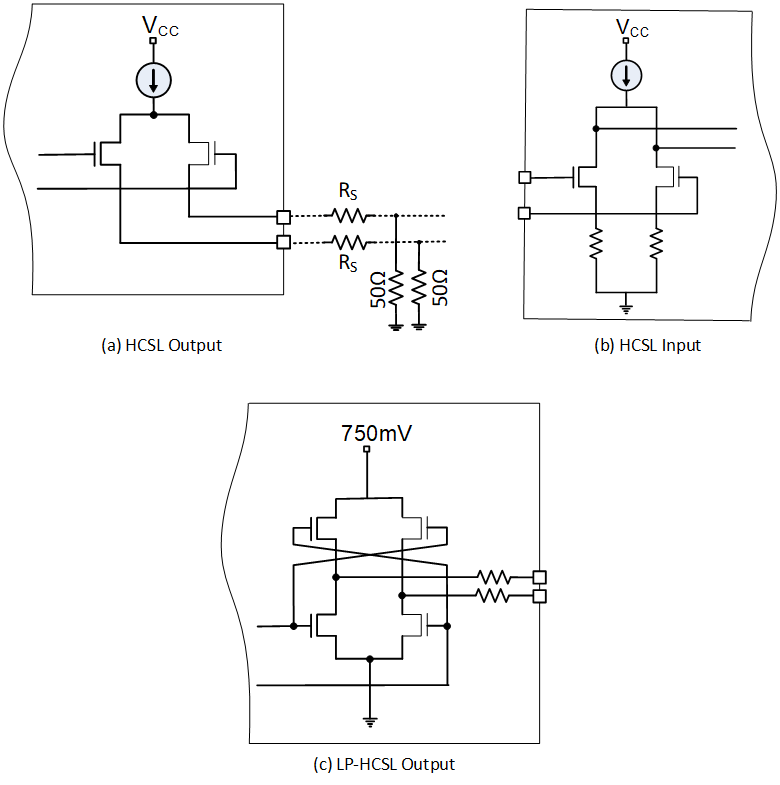The increased demand for high-speed data transmission fueled by social media and online activities in the past two decades lead to the use of more complex ICs operating at higher speed on higher density PCBs. The combination of the high density of a PCB and high-speed signals traveling on it are a good source for interference between different components when interconnecting them.

When dealing with high-speed signaling, interconnects between components must be treated as transmission lines and line termination must be considered to avoid impedance mismatching and line discontinuities, which lead to signal reflections, interference, and performance degradation. This article aims to give an overview of different transmission line termination techniques to interface between devices with similar or different I/O signal formats (LVPECL, LVDS, CML, HCSL, LP-HCSL). Proper line termination should maintain impedance matching and proper biasing for higher performance and good noise immunity and provide the right signal translation to avoid I/O incompatibilities, which can lead to device malfunction, eventual reliability issues and—in the worst case—device damage.

DC coupling vs AC coupling

When DC coupling a driver to a receiver, both the continuous and switching components of the signal will flow from the driver output to the receiver input. While in AC coupling, only the switching component of the signal will reach the receiver since the continuous component will be blocked by the coupling capacitor.

DC coupling offers the advantage of less components count and less power consumption over AC coupling. However, with  DC coupling devices, compatibility between driver’s output and receiver’s input is not always guaranteed and, in some cases, comes with the price of adding more components with increase in power consumption. In many cases DC coupling is not possible at all, leaving AC coupling as the only solution.

AC coupling blocks the DC component of the signal between the driver’s output and the receiver’s input thus eliminating the issue of common mode voltage incompatibility between them. The receiver’s input can then be biased at the optimum levels that offers the best performance in terms of jitter, duty cycle distortion, and crossing. While there is no issue with AC coupling clock signals, AC coupling data signals requires that the data be DC-balanced (same overall number of zeros and ones). This will avoid signal decay in the absence of transitions (during long chains of identical bits) and at the two ends of the receiver termination to the same level, which will reduce noise margin.

To understand the driver receiver compatibility, let’s look at Figure 1. In this example, the driver’s output and the receiver’s input have the same common mode voltage and the driver’s output signal levels fall within the receiver’s input signal level range.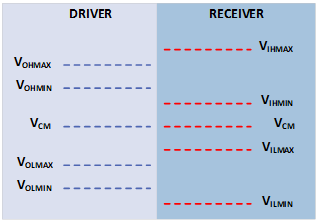Figure 1 The driver’s output and the receiver’s input voltage level  which have the same common mode voltage where the driver’s output signal levels fall within the receiver’s input signal level range. Source: Microchip

This is the case when interfacing devices with the same I/O format, especially when they are from the same manufacturer. It is the optimal configuration for DC coupling between the two devices. This perfect matching is not always offered and sometimes even interfacing devices with the same I/O format from different manufacturers requires special care when DC coupling. When the gap between the common mode voltage of the receiver’s input and the common mode of the driver’s output is large enough to cause the driver’s signal to go beyond the receiver input range. This results in DC coupling incompatibility and AC coupling must be used to keep the driver and receiver at their sweet spots of operation. Figure 2 shows I/O operating levels of commonly used format in high-speed interconnect, LVPECL, LVDS, CML, and HCSL.Figure 2 I/O operating levels of commonly used format in high-speed interconnect, LVPECL, LVDS, CML, and HCSL. Source: Microchip

I/O structures

To understand how to interface between different driver/receivers, let’s overview the I/O structure for the most common logics used for ICs interfacing LVPECL, LVDS, CML, HCSL.

As shown in Figure 3, the LVPECL output stage consists of a differential pair driving an emitter follower pair. The output should be terminated with 50Ω to VCC-2V to create a common mode voltage of VCC-1.3V at the output corresponding to 14mA current flowing through the 50Ω. The output can also be terminated with a Thevenin network (130Ω to VCC / 82Ω to GND) or just a 100Ω to 200Ω resistor to GND. The PECL input stage consists of a switching differential pair that sometimes integrates a high impedance bias resistor network.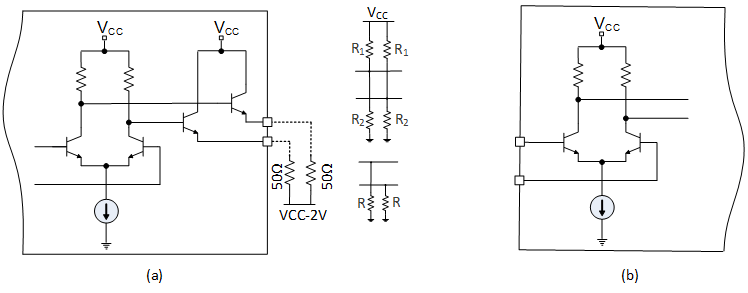Figure 3 The (a) PECL output stage consists of a differential pair driving an emitter follower pair and (b) PECL input stage consists of a switching differential pair that sometimes integrates a high impedance bias resistor network. Source: Microchip

The LVDS output consists of a current-mode driver which sources 3.5mA through a switching network to the differential output (Figure 4). The output is usually connected to a 100Ω differential transmission line which requires a 100Ω differential termination at the receiver side to match the transmission line and create the 350mV swing. The standard common mode for LVDS is 1.2V regardless of VCC. LVDS input stage consists of a switching differential pair with or without an integrated 100Ω resistor to terminate the driver output.Figure 4 The (a) LVDS output consisting of a current-mode driver which sources 3.5mA through a switching network to the differential output and an (b) LVDS input stage consisting of a switching differential pair with or without an integrated 100Ω resistor to terminate the driver output. Source: Microchip

The CML output stage consists of a differential pair of common-emitter transistors with a 16mA switching current and a 50Ω collector resistance to VCC (Figure 5). This results in a 400mV swing (from VCC to VCC-400mV) and a common mode voltage of VCC-200mV. The CML input structure consists of common emitter pair driving a differential pair with or without integrated 50Ω termination to VCC at the input. If not integrated, the 50Ω must be installed on the PCB.Figure 5 The (a) CML output stage consists of a differential pair of common-emitter transistors with a 16mA switching current and a 50Ω collector resistance to VCC and a (b) CML input stage consists of common emitter pair driving a differential pair. Source: Microchip

The HCSL output (Figure 6) consists of a differential pair with open source which steers a 15mA constant current between the true and complementary output. The circuit requires an external 50Ω termination to ground to create the 750mV swing and a series resistor to increase the driver’s output impedance (about 17Ω) to the transmission line characteristic impedance (50Ω). The HCSL input is a differential pair that can accept 700mV at each input and has standard common mode voltage of about 350mV. Finally, the LP-HCSL output stage consists of a push-pull voltage drive stage powered from a 750mV voltage source. No external 50Ω to ground termination needed as in HCSL. The series resistor can be integrated inside the chip to minimize external components count.Figure 6 The (a) HCSL output consists of a differential pair with open source, the (b) HCSL input differential pair, and the (c) LP-HCSL output with a of a push-pull voltage drive stage powered from a 750mV voltage source. Source: Microchip

DC coupling LVDS driver

For DC coupling from the LVDS driver to the LVDS receiver, just connect the LVDS output to the LVDS input (Figure 7) and if the receiver doesn’t have internal termination, terminate with external 100Ω differential close to the receiver input.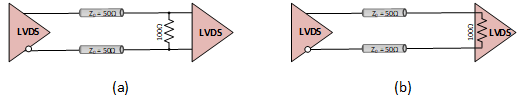Figure 7 LVDS receiver (a) without internal termination and (b) with internal termination. Source: Microchip

The circuit in Figure 8 will work fine for DC coupling from the LVDS driver to the LVPECL receiver even though the difference between the common mode voltage, 1.2V for LVDS vs VCC-1.3V for LVPECL, this is due to the wide common mode range of the LVPECL input and the relatively small swing of LVDS (400mV) which will not cause the saturation of the LVPECL input stage current source.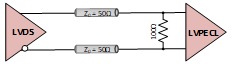Figure 8 DC coupling LVDS driver to LVPECL receiver. Source: Microchip

Another solution to DC couple LVDS to LVPECL is to use a resistor network to shift the DC level from LVDS common mode voltage (1.2V) to LVPECL common mode voltage (VCC-1.3V). This can be achieved using the circuit in Figure 9.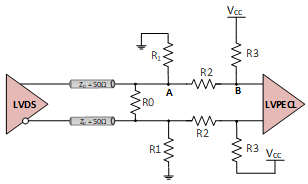Figure 9 DC coupling LVDS to LVPECL by level shifting. Source: Microchip

Resistors values can be calculated from the following equations dictated by the following circuit constraints.

LVDS common mode voltage at point A:

(R1/(R2+R3)) Vcc = 1.2       (1)

LVPECL common mode voltage at point B:

((R1+R2)/(R1+R2+R3)) Vcc = Vcc-1.3       (2)

Impedance matching:

(R0/2) // (R1 // (R2+ R3) = 50       (3)

Considering Vcc = 3.3 V and solving (1) and (2) leads to R2 = 0.615 R3 and R1 = 0.571 (R2+R3).

For R2=200 Ω, R3=325 Ω (324 Ω normalized), and R1 = 299 Ω (301 Ω normalized).

Equation (3) leads to R0 = 136 Ω (137 Ω normalized).

Selecting high-value resistors has the advantage of low-power consumption while lower value resistors allow the circuit to perform better at higher frequencies.

Finally, due to the large gap between the LVDS and CML common mode voltage it’s not practical to DC couple LVDS driver to CML receiver and vice versa.

DC coupling LVPECL driver

For DC coupling the LVPECL driver to the LVPECL receiver, the conditions for proper biasing and impedance matching allow us to calculate the values of the components in Figure 10.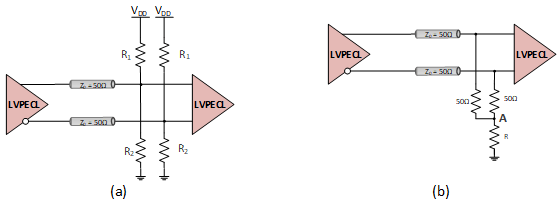Figure 10 DC coupling the LVPECL driver to the LVPECL receiver where the conditions for proper biasing can be calculated with the equations (4) through (6). Source: Microchip

In Figure 10-a the Thevenin termination is equivalent to the 50Ω to VCC-2V standard LVPECL termination and satisfy equations (4) and (5):

R1.R2 / (R1 + R2) = 50       (4)

R2 / (R1+R2). Vcc = Vcc – 2       (5)

R1 and R2 solutions for these two equations are:

R1 = 50.Vcc / (VCC-2)

R2 = 25.Vcc

For Vcc = 3.3 V, R1 = 127 Ω and R2 = 82.5 Ω.

In Figure 10-b, the voltage at node A is VCC-2V (PECL termination: 50Ω to VCC-2V) and the current flowing through R is the sum of the currents flowing through the two 50Ω termination resistors.

I = (VOH-(VCC-2)) / 50 + (VOL-(VCC-2)) / 50        (6)

I = (VOH + VOL -2VCC+4)/50

Thus,

R = (VCC – 2) / I = 50 (VCC-2) / (VOH + VOL -2VCC+4).

If we consider SY58012U as an example, Table 1 shows R values for VCC = 3.3V and 2.5V.

Table 1

VCC

VOH

VOL

R

3.3V

VCC-0.895V

VCC-1.695V

40Ω

2.5V

18Ω

The DC coupling the LVPECL driver to the LVDS receiver can be seen in Figure 11 and the conditions for proper biasing and impedance matching can be solved in equations (7) through (12).Figure 11 Circuit for DC coupling the LVPECL driver to the LVDS receiver (a) without internal termination and (b) with internal termination. Source: Microchip

The voltages at nodes A and B in both Figures 11-a and 11-b are:

A: VCM (LVPECL) = Vcc-1.3V = 2 V

B: VCM (LVDS) = 1.2 V

In Figure 11-a we have:

(R2+R3)/(R1+R2+R3) x 3.3 = 2       (7)

R3/(R1+R2+R3) x 2= 1.2       (8)

R1//(R2+R3) = 50       (9)

The values of R1, R2 and R3 satisfying these equations are R1 = 82.5 Ω, R2 = 51 Ω, and R3 = 75.8 Ω.

In Figure 11-b where the receiver has internal differential 100Ω termination we have:

(R2+R3)/(R1+R2+R3) x 3.3 = 2       (10)

R3/(R1+R2+R3) x 2= 1.2       (11)

R1//(R2+R3//50) = 50       (12)

The values of R1, R2 and R3 satisfying these equations are R1=102Ω, R2=63.4Ω, R3=95.3Ω

It is not recommended to DC couple the LVPECL driver to CML receiver unless AC coupling cannot be used due for example to unbalanced data. In this case the diagram on Figure 12 can be used at the cost of more components and power dissipation. From the LVPECL output, the resistor network is seen as a Thevenin termination with 82.5 Ω to GND and 127 Ω to Vcc (208 // (275+50)). The CML input is biased with the 50 Ω to VCC.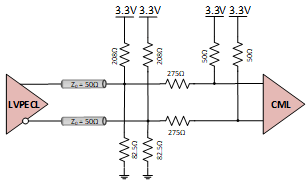Figure 12 DC coupling the LVPECL driver to CML receiver. Source: Microchip

DC coupling CML driver

Due to the high common mode voltage of the CML driver (VCC-200mV), it’s hard if not impossible to DC couple CML driver to other logics (Figure 13).Figure 13 DC coupling CML driver to CML receiver where the driver (a) has an internal termination and (b) does not have an internal termination. Source: Microchip

DC coupling HCSL/LPHCSL driver

The LPHCSL is a voltage driver which doesn’t require the 50 Ω termination to GND necessary for HCSL driver which is a current source that needs a path to ground. Due to the low common mode voltage of the HCSL/LPHCS driver (250 mV-550 mV), it is also hard if not impossible to DC couple HCSL/LPHCSL driver to other logics (Figure 14).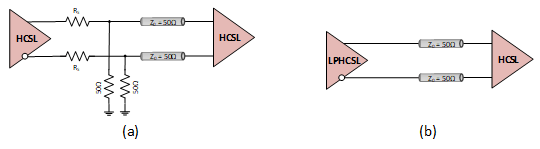Figure 14 DC coupling HCSL driver to HCSL receiver (a) and DC coupling the LPHCSL driver to HCSL receiver (b). Source: Microchip

AC coupling LVDS driver

The AC coupling for the LVDS driver to the LVDS receiver can be seen in Figure 15. For LVDS receivers without internal termination, the termination network on Figure 15-a sets the appropriate termination at the receiver input and sets the LVDS input common mode voltage. If the receiver has internal termination, the external network used to generate the common mode voltage should use high value resistors to preserve the transmission line termination (100 Ω differential). The 5.1K and 9.1K resistors on Figure 15-b set the common mode voltage to 1.2 V.Figure 15 AC coupling LVDS driver to an LVDS receiver (a) without an internal termination and (b) with an internal termination. Source: Microchip

Figure 16 shows the AC coupling for the LVDS driver to the LVPECL, CML, and HCSL receivers. The termination network in Figure 16-a sets the LVPECL input common mode voltage (VCC-1.3V) and provides a 50 Ω line termination (100 differential). If the receiver has a VBB (2 V) bias source, just terminate each input with a 50 Ω to VBB. For Figure 16-b, the 50Ω resistors provide the bias to the CML input and the 100Ω differential termination to the LVDS driver. For AC coupling the LVDS driver to the HCSL receiver in Figure 16-c, the 471Ω/ 56Ω network sets the transmission line termination to 50Ω and HCSL receiver common mode voltage to about 350mV.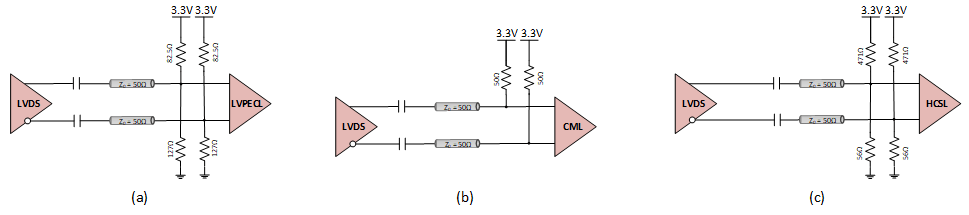Figure 16 AC coupling for the LVDS driver to the (a) LVPECL, (b) CML, and (c) HCSL receivers. Source: Microchip

AC coupling LVPECL driver

Figure 17 shows AC Coupling the LVPECL driver to the LVPECL, LVDS, and CML receivers. In Figure 17-a, the 150 Ω provides a path to ground to the emitter follower and sets the LVPECL output common mode voltage and the 82.5Ω/127Ω network terminates the line with 50 Ω and sets the LVPECL input common mode voltage to VCC-1.3V. In Figure 17-b, the 100 Ω terminates the transmission line and the 5.1KΩ/9.1KΩ network sets the LVDS input common mode voltage. In Figure 17-c, the 50Ω resistor terminates the line and sets the bias for the CML input while the series resistor attenuates the PECL signal to be within range for the CML input. In Figure 17-d, the 471Ω/56Ω network provides a 50Ω line termination and set the HCSL input common voltage close to 400mV.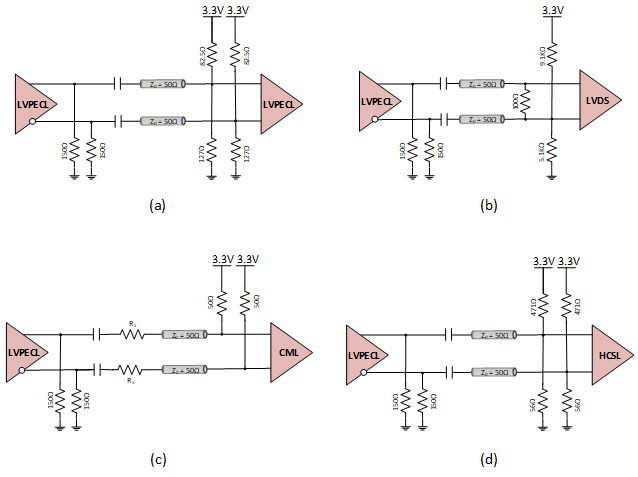Figure 17 AC Coupling the LVPECL driver to the (a) LVPECL, (b) LVDS, (c) CML, and (d) HCSL receivers. Source: Microchip

AC coupling CML driver

As shown in Figure 18, for AC coupling the CML driver to CML receiver, if the driver doesn’t have internal 50Ω termination to VCC, the output must be terminated outside before the coupling cap.Figure 18 AC coupling CML driver to a CML receiver (a) with an internal termination and (b) without an internal termination. Source: Microchip

Figure 19 shows AC Coupling the CML driver to the LVDS, PECL, and HCSL receivers. The CML driver in Figure 19-a has internal 50Ω termination to VCC and LVDS receiver doesn’t have internal termination. The 5.1KΩ/9.1KΩ network sets the 1.2V common mode voltage for the LVDS receiver. For CML driver without internal termination refer to Figure 13 for and LVDS receiver with internal termination refer to Figure 15.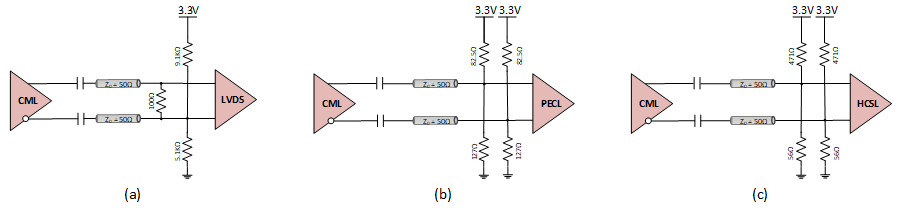Figure 19 AC Coupling the CML driver to the (a) LVDS, (b) PECL, and (c) HCSL receivers. Source: Microchip

AC Coupling HCSL Driver

Figure 20 shows the AC coupling from the HCSL driver to HCSL receiver as well as the LPHCSL driver to the HCSL receiver.Figure 20 AC coupling from the (a) HCSL driver to HCSL receiver and (b) LPHCSL driver to the HCSL receiver. Source: Microchip

Solving for R1 and R2 to set the common mode voltage at the HSCL input to 350mV leads to equation:

R2=R1(Vcc/0,35 – 1)       (13)

To avoid attenuating the signal at the input of the receiver just use high values for R1 and R2. For R1 = 5.1 KΩ, R2 will be 43.2 KΩ. As an example, the values R1 = 56.2 Ω and R2 = 475 Ω can be selected to match the transmission line impedance at the cost of signal swing drop by half. Finally, the AC coupling for the HCSL driver to the LVDS, LVPECL, and CML receivers can be seen in Figure 21.Figure 21 AC coupling for the HCSL driver to the (a) LVDS, (b) LVPECL, and (c) CML receivers. Source: Microchip

Proper ICs interconnect for high speed signaling

To successfully interface between high-speed ICs populating high density boards, it is important to know the specifications of the driver output and receiver input. Only when obtaining these specifications, the transmitted signal type, or clock signal or balanced/unbalanced data can someone decide on the type of coupling to be used (DC or AC). It is important to always use the topology that preserves the integrity of the signal first and then prioritize choosing the less complex topology second, with minimum components and low-power consumption in mind. In this article, we displayed solutions for interfacing between LVDS/LVPECL/CML/HCSL (there may be other circuits weren’t shown). To define a new interface, it is important to always solve for network elements that satisfy the basic constraints that will preserve the transmitted signal integrity. These elements include proper common mode voltage, impedance matching, and signal reaching the receiver input within receiver input range.

Abdennour Mezerreg is a senior technical staff applications engineer for Microchip Technology’s timing and communications business unit.

Related Content

Interconnecting common interfaces
Interfacing LVDS with other differential-I/O types
Understanding LVDS Fail-Safe Circuits
LVDS, CML, ECL-differential interfaces with odd voltages
Survival guide to high-speed A/D converter digital outputs part 2

<!–
–>

The post Proper IC interconnects for high-speed signaling appeared first on EDN.